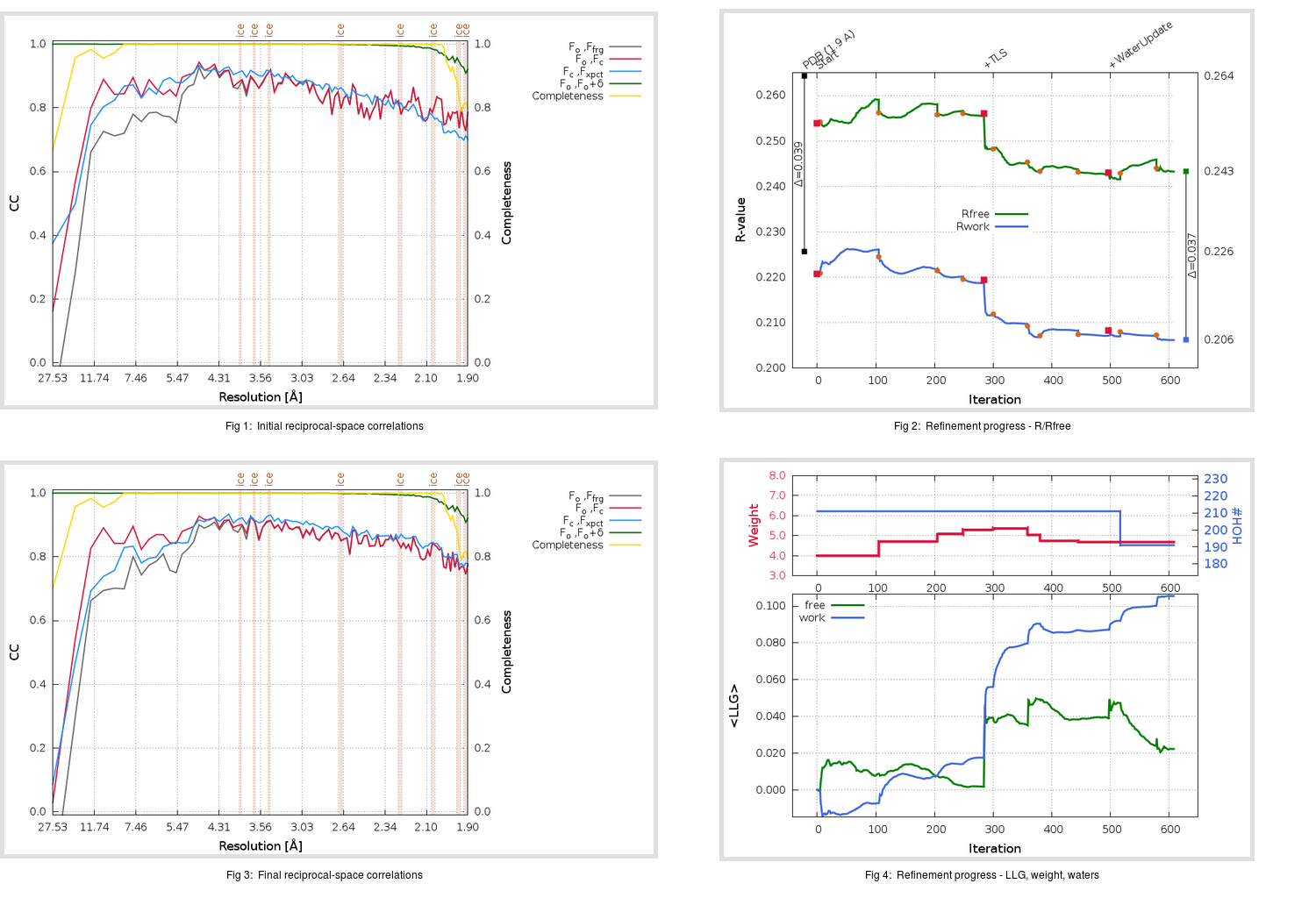Content:

```    Diffraction limits & principal axes of ellipsoid fitted to diffraction cut-off surface:
1.889         1.0000   0.0000   0.0000       a*
1.843         0.0000   1.0000   0.0000       b*
1.880         0.0000   0.0000   1.0000       c*
```

## Deposited

` `
 Date deposited Date data collection Resolution R, Rfree 20051012 20041104 1.90 0.2256 0.2643

Molprobity (CCP4 7.0 version) summary:

```Ramachandran outliers =   1.34 %
favored =  95.65 %
Rotamer outliers      =   3.45 %
C-beta deviations     =     0
Clashscore            =  13.34
RMS(bonds)            =   0.0067
RMS(angles)           =   1.35
MolProbity score      =   2.35
Resolution            =   1.90
R-work                =   0.2256
R-free                =   0.2643
```

```Number of waters      =   211

<B> (all atoms) =   34.77 ( sd =   12.91 ) for       2562 non-hydrogen atoms
<B>   (protein) =   33.90 ( sd =   12.68 ) for       2351 non-hydrogen atoms
<B>     (water) =   44.35 ( sd =   11.16 ) for        211 non-hydrogen atoms
<B>    (others) =    0.00 ( sd =    0.00 ) for          0 non-hydrogen atoms

B min/max       (all non-hydrogen atoms) =   11.40 /   87.71
B min/max   (protein non-hydrogen atoms) =   11.40 /   67.68
B min/max     (water non-hydrogen atoms) =   12.27 /   87.71
B min/max     (other non-hydrogen atoms) =    0.00 /    0.00
```

## BUSTER (re-)refinement

` `

Molprobity (CCP4 7.0 version) summary:

```Ramachandran outliers =   0.67 %
favored =  98.33 %
Rotamer outliers      =   5.36 %
C-beta deviations     =     1
Clashscore            =   3.66
RMS(bonds)            =   0.0117
RMS(angles)           =   1.58
MolProbity score      =   1.71
Resolution            =   1.90
R-work                =   0.2062
R-free                =   0.2432
```

```Number of waters      =   191

<B> (all atoms) =   35.44 ( sd =   12.89 ) for       2542 non-hydrogen atoms
<B>   (protein) =   34.39 ( sd =   12.10 ) for       2351 non-hydrogen atoms
<B>     (water) =   48.17 ( sd =   14.97 ) for        191 non-hydrogen atoms
<B>    (others) =    0.00 ( sd =    0.00 ) for          0 non-hydrogen atoms

B min/max       (all non-hydrogen atoms) =   12.37 /  149.22
B min/max   (protein non-hydrogen atoms) =   12.37 /   75.16
B min/max     (water non-hydrogen atoms) =   17.14 /  149.22
B min/max     (other non-hydrogen atoms) =    0.00 /    0.00
```

Refinement progression:Results:

` `
 File Remark 2C3S_aB_refine.01_03_refine.pdb.gz exact refinement commands are in header 2C3S_aB_refine.01_03_refine.mtz.gz including original deposited data and several re-refinement map coefficients 2C3S_aB_refine.01_03_BUSTER_model.cif.gz including any non-standard compound restraints 2C3S_aB_refine.01_03_BUSTER_refln.cif.gz